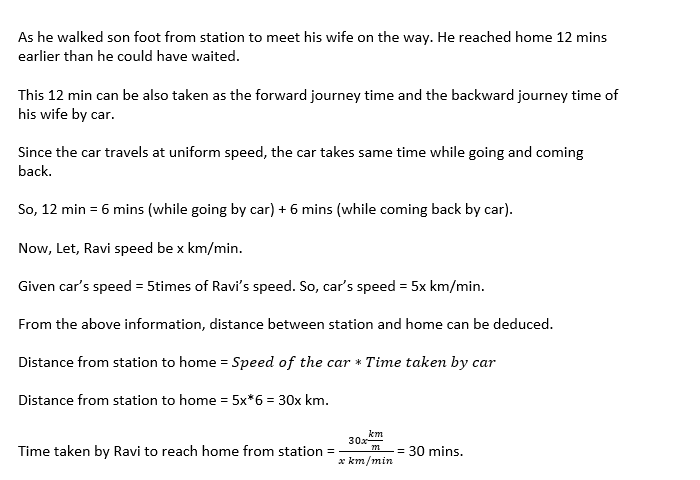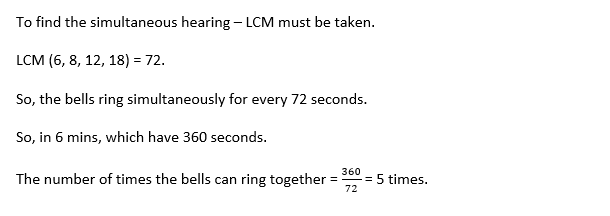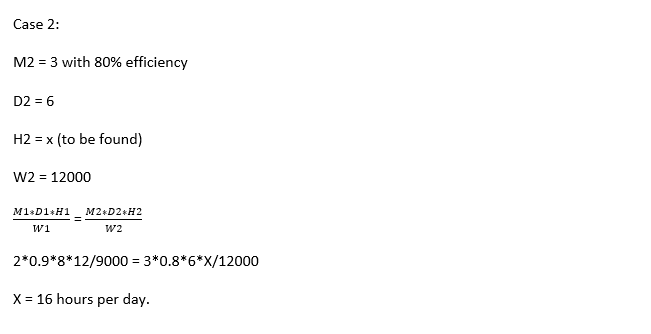# Dell Quantitative Aptitude Previous Year Questions

11 January 2023

Dell Quantitative Aptitude Previous Year Questions

Dell Quantitative Aptitude Previous Year Questions

Q1) Amar caught an earlier train to home today than which he usually takes. His wife normally drives down in a car to pick him up from the station. Today he set out on foot from the station to meet his wife on the way. He reached home 12 minutes earlier than he would have done had he waited for his wife. The car can travel at a uniform speed that is 5 times Amar’s speed on foot. Amar reached home as the clock was striking 6 o’clock (pm).

What time would Amar have reached home, if his wife, forewarned of his change of plans, had meet him at the station?

A.5:50 pm

B.5:30 pm

C.5:36 pm

D.None of these

Explanation:Q2) A gentleman starts at 7 km/hr to go to his native place. A messenger started 30 min later, follows him and overtakes him after he has gone over 17 ½ km. At what rate did the messenger follow the gentleman?

A.7 ¾ km/hr

B.5 ¾ km/hr

C.6 ¾ km/hr

D.8 ¾ km/hr

Explanation: Speed of gentleman = 7km/hr

Distance travelled by gentleman before messenger overtakes = 17 ½  km

Time for which gentleman was ahead = Distance ÷ Speed

Time for which gentleman was ahead = 17 ½ /7 hr = 2.5 hr

Time for which messenger travelled = 17 ½ /7 hr - 30 min = 2 hr

Distance travelled by messenger = Distance travelled by gentleman

Speed of messenger =   17.5/2 km/hr = 8.75 km/hr = 8 ¾ km/hr.

Q3) The ratio of the age of a man and his wife is 4 : 3. After 4 years, this ratio will be 9 : 7. If at the time of the marriage, the ratio was 5: 3, then how many years ago they were married?

A.8 years

B.12 years

C.10 years

D.15 years

Explanation: Let their present age be 4x & 3x.

Then, after 4years the husband's age =4x+4 and the wife's age =3x+4.

So, by the given condition,

(4x+4)/ (3x+4)?) = 9/7?

28x+28 = 27x+36

x=8.

Then the present age of the husband=4×8years=32years &

the wife's age=3×8years=24years.

Let their marriage took place P years back.

Then, by the given condition,

32−P /24−P ?=5/3

96−3P=120−5P

2P=24

P=12yrs.

Q4) Arti and Barkha start swimming towards each other from the deep end and shallow end respectively of a swimming pool in Funcity. They start their swimming simultaneously in the pool of 300 m length. The ratio of their speeds is 1:2 respectively. Each swimmer rests for 6 seconds once she reaches the other end and starts swimming back. Where will they meet for the second time in the still water of swimming pool?

A.At the shallow end

B.At the deep end

C.Can’t be determined

D.30 m from the shallow end

Explanation:

Shallow end (Barkha)                                                                 Deep end (Arti)

Given ratio of speeds = 1 : 2

So, let the speed of Arti be 1 m/s

The speed of Barkha be 2 m/s.

Length of the pool = 300 m

Time taken by Arti from Deep to shallow end (1st meet) = 300/1 = 300 sec + 6 sec of break = 306 secs

Now, time taken by Barkha from Shallow to deep end (1st meet)  = 300/2 = 150 sec + 6 second of break = 156 seconds.

Now, Barkha reached the other end and start to her initial point (shallow end) = another 150 sec.

So, totally Barkha takes 306 seconds time to reach her first point, which is the shallow end.

At 306th seconds both of them meet for the second time at the shallow end. So, answer is option A.

Q5) In a certain state, the army was originally organized in battalions, each of 1500 men. After calling up a further 10000 recruits the army was rearranged so that there were 2000 men in each battalion and the state claimed that it had reduced its army by 50 battalions. What was the number of battalions originally?

A.440

B.220

C.110

D.330

Explanation:

Let initial number of battalions =Let initial total number of recruits =Each battalion has 1500 number of men.

So, as per question statement:

Each battalion has 1500 number of men.

So, according to the given information,

1500x = y

Given that there are 10000 new recruits,

So, total number of recruits = y  + 10000

New number of men in each battalion = 2000

There are 50 battalions that were reduced = x – 50 battalions are there.

New number of recruits = 2000 (x-50)

And the equation becomes,

Y + 10000 = 2000 (x-50)

So, original number of battalions, X = 220.

Q6) An employer reduces the number of his employees in the ratio 9 : 8 and increases their wages in the proportion 14 : 15 .The difference in wage bill if it was previously Rs. 1890, will be

A.Rs. 90 more

B.Rs. 90 less

C.Rs. 100 less

D.Rs. 180 more

Explanation:

Employees * wage = Expenditure

Old : New

Employees       9    :    8

Wage               14   :    15

Expenditure = 126 : 120

126 parts = 1890.

Each part = 15 parts.

The difference of expenditure is 6 parts.

So, 6 parts = Rs. 90.

Q7) ‘A’ sells goods at the price marked on them. ‘B’ sells at 5% less than the price marked. Actually ‘B’ sells for Rs. 1960, the goods that are sold in ‘A’ for Rs. 2000. What price does ‘B’ mark on an article that is sold in ‘A’ for Rs. 190?

A.Rs. 225

B.Rs. 196

C.Rs. 159

D.Rs. 175

Q8) Four bells are heard at intervals of 6, 8, 12 and 18 seconds respectively since 12 o’clock. How many times can they be heard simultaneously within 6 minutes of the time (excluding the one at the start)?

A.8

B.6

C.9

D.5

Explanation:Q9) Two coal loading machines each working 12 hours per day for 8 days handles 9 tons of coal with an efficiency of 90%. While 3 other coal loading machines of an efficiency of 80% set to handle 12 tons of coal in 6 days. Find how many hours per day each should work?

A.16 hrs/day

B.12 hrs/day

C.20 hrs/day

D.18 hrs/day

Explanation: Case 1:

M1 = 2 with 90% efficiency

D1 = 8

H1 = 12

W1 = 9000Q10) If 1 gm of gold 10 carats fine, 1 gm of gold 11 carats fine, 2 gm of gold 12 carats fine, and 5 gm of gold 13 carats fine be mixed together, then the fineness of the resulting compound is

A.12.22 carats

B.12.00 carats

C.12.50 carats

D.12.70 carats

Explanation: We have been given that

1gm of gold 10 carats fine;

1gm of gold 11 carats fine;

2gm of gold 12 carats fine;

5gm of gold 13 carats fine;

These all are mixed together; we have to find the Fineness of the resulting compund.

Total grams of gold = 1+1+2+5=9gms

Fineness=10×1/9+11×1/9+12×2/9+13×5/9

Fineness=12.23grams

Q11) A boy wishes to cut as many pieces of rope of equal length as he can from pieces that are 104, 78 and 65 m long. If he wants the pieces to be as long as possible and does not like to waste any rope, then how long should each piece be?

A.15

B.16

C.13

D.18

Explanation: The length of 1st rope = 10478 m and the length of 2nd rope = 65 m.

Take the HCF of 10478 m and 65 m.

the HCF of 10478 and 65, we have

10478 = 2×13×13×31

65= 5×13

the HCF of 10478 and 65 =13

thus, 13 m of rope should be cut from the given ropes.

Q12) The weights of three lumps of metal are in the ratio 3 : 4 : 5. By what fractions of themselves must the first two be increased so that the ratio of the weights may be changed to 5 : 4 : 3?

A.3/4, 4/3

B.9/16, 3/2

C.2/3,1/4

D.16/9, 2/3

Explanation: Given in the question, Initial weight ratio = 3      :      4      :      5

|+x         | +y        |(no change)

first two increments, the new weight         = 5      :      4      :      3.

Since the third metal did not change, let us make them in same parts.

So, multiplying the first ratio with 3 and second with 5.

The new ratios are 9 : 12 : 15 & 25 : 20 : 15.

So, the first metal is increased by 16/9 and the second by 8/12 = 2/3.

So, option D is the answer.

Q13) A man sells sugar to a tradesman at a profit of 20%, but the tradesman, becoming bankrupt, pays only 72 paise per rupee. How much percent does the man gain or lose by his sale?

A.15% profit

B.13.6% loss

C.12% profit

D.86.4% loss

Explanation: Let, Cost Price of Sugar = 100

Profit of 20 % = (20/100) *100 = 20

Selling Price = 120

Received 72 paise per rupee = (72/100) 120 = 86.4

Loss = 100 - 86.4 = 13.6

Loss % = (13.6/100C) * 100 = 13.6 %

13.6 % Loss

Q14) The average monthly income of three persons is Rs. 3,600. If the income of the first is 1/5 of the combined income of the other two then his monthly income is:

A.None of these

B.Rs. 2400

C.Rs. 1500

D.Rs. 600

Explanation: Let three persons income be A, B and C respectively.

Given A + B + C = 3600.

And also given, A = 1/5 (B + C)

B + C = 5A.

Then A + 5A = 3600.

6A = 3600.

A = 600.

So, his (first person’s) income = 600.

Q15) The average age of 30 students in a class is 16 years. If the age of their teacher is 47 years. What is the average age of the students and the teacher combined?

A.19 years

B.17 years

C.25 years

D.30 years

Explanation: As the average age of 30 students is 16 years,

therefore, sum of the age of those 30 students = 30 × 16 years = 480 years

Now, if the age of their teacher is combined with their age,

Then the sum of the age of 31 persons = 480+47=527 years

Therefore, the average of their age is 527÷31=17 years

Hence, the average age of the students and the teacher combined is 17 years.

##